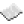# Difference between revisions of "Window.reposition"Function window.reposition A function available to window-based terminal objects created via the window API, which moves and / or resizes the window in concern. If visible, the window will be automatically re-rendered, however the parent terminal object won't be (meaning "ghost" images of the window's previous shape / location may be left behind unless you manually clear them). If shrinking a window will result in some of its contents being cropped from view, then that content will be removed from the window's buffer and will not be restored if you later re-enlarge it. If a new width / height is not specified then the window will retain its previous dimensions. See also: window.getPosition(), term.getSize() Syntax window.reposition(number xPosition, number yPosition [, number width, number height]) Returns None Part of ComputerCraft API termExample Stretches and bounces a small window filled with white around the display. Code ```local xSize, ySize = term.getSize() local xPos, yPos, xLen, yLen = math.random(xSize-5), math.random(ySize-5), math.random(5), math.random(5) local xDir, yDir, xLenDir, yLenDir = 1, 1, 1, 1 local smallWindow = window.create(term.current(), xPos, yPos, xLen, yLen) smallWindow.setBackgroundColor(colours.white) while true do sleep(0.2) xPos = xPos + xDir yPos = yPos + yDir xLen = xLen + xLenDir yLen = yLen + yLenDir term.setBackgroundColor(colours.black) term.clear() smallWindow.reposition(xPos, yPos, xLen, yLen) smallWindow.clear() if xPos <= 1 then xDir = 1 end if xPos + xLen >= xSize + 1 then xDir = -1 end if yPos <= 1 then yDir = 1 end if yPos + yLen >= ySize + 1 then yDir = -1 end if xLen <= 1 then xLenDir = 1 end if xLen >= 5 then xLenDir = -1 end if yLen <= 1 then yLenDir = 1 end if yLen >= 5 then yLenDir = -1 end end ```